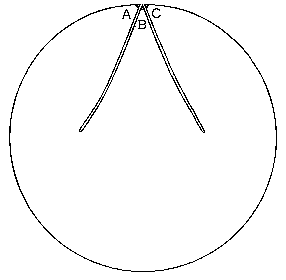﻿ Wiggly Triangles Solutions < Back to the problem
Home

# Solutions for the Right Wiggly Triangles

## Part 1

Suppose there are
• t right wiggly triangles in the circle
• p points inside the circle but not on the outside where the triangles meet
• q points on the circle itself (and therefore also q lines)
• e edges inside the circle - not counting the q on the outside
Then we have:
• Number of faces = t+1 including the outside
• Number of edges = e+q
• Number of vertices = p+q
Firstly add up the angles of the triangles. It is 180° per triangle. Also it is 360° per interior point and 180° for each on the circle.
180t = 360p + 180q
or simplifying
t = 2p + q
Now count the total number of sides of the triangles. Each interior side is on two triangles whereas those on the circle are only on one triangle.
3t = 2e + q
Subtracting the previous equation t=2p+q from this gives;
2t = 2e - 2p
Which is the same as
t + p = e
However by Euler's formula F+E=V+2 therefore
t+1 + p+q = e+q + 2
which gives
t + p = e + 1
and subtracting t+p=e from this gives the classical contradiction
0 = 1
QED we can't divide up a circle into right wiggly triangles, it is just plain impossible.

## Part 2

You'll like this (not a lot!).

A single right wiggly triangle is sufficient. The sides AB and BC are bent back on themselves so corners A, B and C all meet at a point. AC forms the outside of the circle. Angles A+B+C make up 180°.